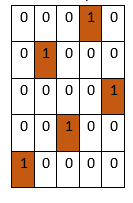# Program to check whether a board is valid N queens solution or not in python

PythonServer Side ProgrammingProgramming

Suppose we have a n x n matrix represents a chess board. There are some 1s and 0s, where 1 represents a queen and 0 represents an empty cell. We have to check whether the board is valid solution to the N-Queen puzzle or not. As we know a board is a solution of valid N-queen solution where no two queens are attacking each other.

So, if the input is likethen the output will be True

To solve this, we will follow these steps:

• n := row count of matrix
• rows := a new set, cols := a new set, diags := a new set, rev_diags := a new set
• for i in range 0 to n, do
• for j in range 0 to n, do
• if matrix[i, j] is 1, then
• insert i into rows
• insert j into cols
• insert (i - j) into diags
• insert (i + j) into rev_diags
• return true when size of rows, size of cols, size of diags, size of rev_diags is same as n, otherwise false

Let us see the following implementation to get better understanding:

## Example

Live Demo

class Solution:
def solve(self, matrix):
n = len(matrix)

rows = set()
cols = set()
diags = set()
rev_diags = set()

for i in range(n):
for j in range(n):
if matrix[i][j]:

return len(rows) == len(cols) == len(diags) == len(rev_diags) == n

ob = Solution()
matrix = [
[0, 0, 0, 1, 0],
[0, 1, 0, 0, 0],
[0, 0, 0, 0, 1],
[0, 0, 1, 0, 0],
[1, 0, 0, 0, 0]
]
print(ob.solve(matrix))

## Input

matrix = [
[0, 0, 0, 1, 0],
[0, 1, 0, 0, 0],
[0, 0, 0, 0, 1],
[0, 0, 1, 0, 0],
[1, 0, 0, 0, 0]
]

## Output

True